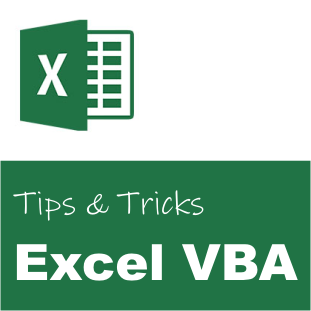# Excel VBA: Convert a Variant Array to a String Array# Excel VBA: Convert a Variant Array to a String Array

To simplify the conversion of a variant array to a string array in Microsoft Excel we have made a set of utility functions. The method will also flatten any variant arrays within the source array (i.e. jagged arrays).

```' Array Variant to String
Public Function VariantArrayToStringArray(ByVal arrVariants As Variant) As String()
Dim arrStrings() As String

' Get the string array
Call ParamArrayToStringArray(arrVariants, arrStrings)

' Get the string array
VariantArrayToStringArray = arrStrings
End Function

' Array Variant to String
Public Sub ParamArrayToStringArray(ByVal arrVariants As Variant, ByRef arrStrings() As String)
Dim intLength As Integer

' Handle the array
Call ParamArrayToStringArrayInternal(arrVariants, arrStrings, intLength)
End Sub

' Array Variant to String
Private Sub ParamArrayToStringArrayInternal(ByVal arrVariants As Variant, ByRef arrStrings() As String, ByRef intLength As Integer)
' Parameter is array?
If (IsArray(arrVariants)) Then
Dim i As Integer
Dim objValue As Variant

' Walk through the specified partner objects
For i = LBound(arrVariants) To UBound(arrVariants) Step 1
' Get the value
objValue = arrVariants(i)

' Array to string
Call ParamArrayToStringArrayInternal(objValue, arrStrings, intLength)
Next
Else
' Next item
intLength = intLength + 1

' Expand array
ReDim Preserve arrStrings(1 To intLength)

' Set the value
arrStrings(intLength) = CStr(arrVariants)
End If
End Sub

' String Array
' Convert ParamArray to String array
Public Function StringArray(ParamArray arrValues() As Variant) As String()
' Get the string array
StringArray = VariantArrayToStringArray(arrValues)
End Function
```

## Usage

Here is an example on how to use the method called VariantArrayToStringArray:

```' Source array
Dim arrVariants() As Variant

' Set the array length
ReDim arrVariants(1 To 4)

' Set the array values
arrVariants(1) = 1          ' Integer
arrVariants(2) = 2.2        ' Single
arrVariants(3) = "Text"     ' Text
arrVariants(4) = True       ' Boolean

' Destination array
Dim arrStrings() As String

' Convert variant array to string array
arrStrings = VariantArrayToStringArray(arrVariants)```

The method will return an array with the following string values:
arrStrings(1) = “1”
arrStrings(2) = “2.2”
arrStrings(3) = “Text”
arrStrings(4) = “True”

Here is an example on how to use the function StringArray to create a string array from a ParamArray of variants. It is a fast way to initialize an array of strings.

```' Destination array
Dim arrStrings() as String

' Get string array
arrStrings = StringArray("This", "is", "a", "test")```

The array arrStrings now contains the following values:

arrStrings(0) = “This”
arrStrings(1) = “is”
arrStrings(2) = “a”
arrStrings(3) = “test”

### Regional settings

Please notice that the decimal separator for singles/floats/doubles is dependent on your computer’s regional settings. In some regions the decimal separator is a comma. For item 2 the result value is “2.2” in the USA, while in the Scandinavian countries the result value is “2,2”.

To always use the dot as a decimal separator, replace the expression CStr(arrVariants) with Trim(Str(arrVariants)). In some cases I have experienced that the Str function adds a space to the result string. This space can be removed by Trim. The Str function does unfortunately not support a string as an input value, so we need to do an additional check.

```' Contains decimal numbers?
If (VarType(arrVariants) <> vbString) Then
arrStrings(intLength) = Trim(Str(arrVariants))
Else
' Convert String to String
arrStrings(intLength) = CStr(arrVariants)
End If```

The full source code for the modified method ParamArrayToStringArrayInternal is shown below.

```' Array Variant to String
Private Sub ParamArrayToStringArrayInternal(ByVal arrVariants As Variant, ByRef arrStrings() As String, ByRef intLength As Integer)
' Parameter is array?
If (IsArray(arrVariants)) Then
Dim i As Integer
Dim objValue As Variant

' Walk through the specified partner objects
For i = LBound(arrVariants) To UBound(arrVariants) Step 1
' Get the value
objValue = arrVariants(i)

' Array to string
Call ParamArrayToStringArrayInternal(objValue, arrStrings, intLength)
Next
Else
' Next item
intLength = intLength + 1

' Expand array
ReDim Preserve arrStrings(1 To intLength)

' Contains decimal numbers?
If (VarType(arrVariants) <> vbString) Then
arrStrings(intLength) = Trim(Str(arrVariants))
Else
' Convert String to String
arrStrings(intLength) = CStr(arrVariants)
End If
End If
End Sub```

## Simplified variant

One of our readers, Pete, proposed a simpler version of the above function (see the comments field below). This variant, however, does not handle jagged arrays, i.e. arrays inside arrays. The function is included for your reference:

```' Converts Var array to a string array
Public Function Variant2String(ByRef vArr() As Variant) As String()
Dim i As Long
Dim sArr() As String
ReDim sArr(LBound(vArr) To UBound(vArr)) As String

For i = LBound(vArr) To UBound(vArr) Step 1
sArr(i) = CStr(vArr(i))
Next

Variant2String = sArr
End Function```

## Compilation

It is recommended to add the expression Option Explicit at the beginning of your source file to ensure that the code is compiled correctly.

## Related##### Ulf Emsoy
Ulf Emsoy has long working experience in project management, software development and supply chain management.

## 6 thoughts on “Excel VBA: Convert a Variant Array to a String Array”

1.ScottD says:

Doesn’t seem to work. Get “Type mismatch” compile error with the following:
Dim msg as string (or as string(60) – either way. Then:
msg = VariantArrayToStringArray(Listarray)
where Listarray is a variant array filled with values from a named range.
Can’t believe it’s so difficult to convert a variant() to a string()!

1.Coragi says:

Thank you for your comment. I have now updated the article with an example.

The destination string array should be declared as:
Dim msg() As String

2.Pete says:

Function Variant2String(vArr() As Variant)
‘converts Var array to a string array
Dim i As Long, sArr() As String
ReDim sArr(UBound(vArr)) As String
For i = 1 To UBound(vArr)
sArr(i) = CStr(vArr(i))
Next

Variant2String = sArr

End Function

1.Ulf Emsoy says:

Thank you for the short version of the function. However, this function does not handle jagged arrays, i.e. arrays in arrays.